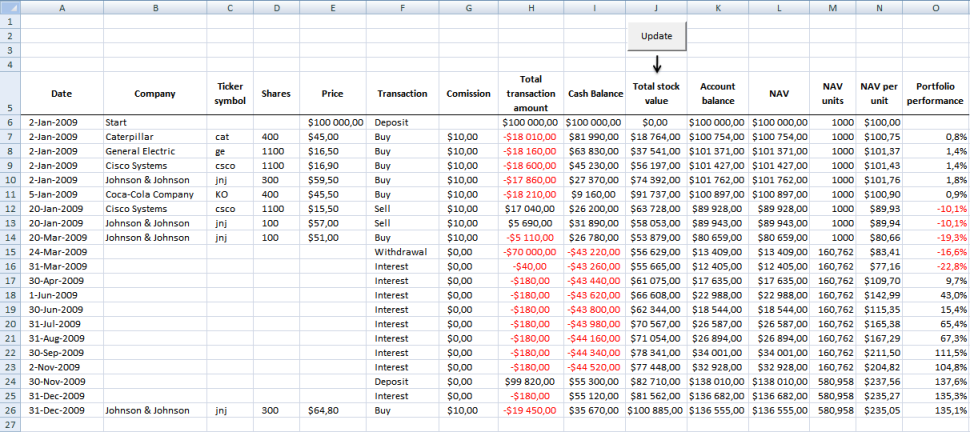Author: Oscar Cronquist Article last updated on August 06, 2017

In a previous related post we calculated the stock portfolio performance using the most current stock prices compared to buying prices. Dividends, interest, deposits and withdrawals are not calculated in this post.

In another previous related post we created a web query to calculate Net Asset Value at any given date. Net Asset Value is cash balance in your stock account + total portfolio stock value. Dividends payed, interest earned and commissions are calculated and affects account balance. Deposits and withdrawals are not calculated in this post.

In this post we are going to calculate units of Net Asset value. Doing so we can easily calculate stock portfolio performance also when a deposit or withdrawal is made. Calculate portfolio performance by comparing your account balance day by day is wrong.  Why? When you make a deposit or withdrawal, portfolio performance is distorted.

Example,

Day 1, Deposit \$10 000 - Account balance: \$10 000

Day 2, Buy 200 shares Caterpillar @ \$40

Account balance: \$9 990 (\$40*200= \$8000 + cash \$2000 - Commission:\$10 = \$9 990)

Day 3, Deposit \$10 000

Account balance: \$9 990+\$10 000= \$19 990 (Caterpillar is still at \$40)

The account balance has increased from Day 1: \$10 000 to Day 3: \$19 990 but the stock price is the same.

Portfolio performance: \$19 990/\$10 000 -1= +99,9%

Clearly calculating stock portfolio performance based on account balance is wrong.

Example - Net asset value based on unitsDay 1, Deposit \$10 000 - Account balance: \$10 000.

I have chosen 100 NAV units in this example but any number will do. NAV / Units is \$10 000 / 100 units = \$100 per unit.

NAV/unit is the number we compare day by day to calculate portfolio performance.

Day 2, Buy 200 shares Caterpillar @ \$40 (Commission \$10) - Account balance: \$9 990

NAV is now \$9 990 and we divide with 100 NAV units equals \$99,90 per unit.

Portfolio performance: 99.90/100.00 -1= -0,1%

Day 3, Deposit \$10 000  - Account balance: \$9 990+\$10 000= \$19 990 (Caterpillar is still at \$40)

Account Balance = NAV. NAV is now \$19 990.

NAV units increases when we do a deposit and decreases when we do a withdrawal.

NAV units increases \$10 000/\$99,90 = 100,1001001 units to a total of 200,1001001 units.

NAV / Units = \$19 990 / 200,1001001 = \$99,90

Portfolio performance: \$99,90/\$100,00 -1= -0,1%

(The commission is affecting stock portfolio by -0,1%.)

Excel tutorial file

In a previous related post we created a web query to calculate Net Asset Value at any given date. I have added NAV units, NAV / units and portfolio performance to the attached file.

Track-your-stock-portfolio-performance.xls

Excel 97-2003 *.xls

Remember to enable macros and you can´t undo a macro. Backup!NAV formula in L7:

=N7*M7 + ENTER

Copy and paste cell down as far as needed.

NAV units formula in M7:

=M6-(F7="Withdrawal")*(-H7/((I7+J7-H7)/M6))+(F7="Deposit")*(H7/((I7+J7-H7)/M6)) + ENTER.

Copy and paste cell down as far as needed.

NAV per unit formula in N7:

=(I7+J7)/M7 + ENTER.

Copy and paste cell down as far as needed.

Portfolio performance formula:

=N7/\$N\$6-1 + ENTER.

Copy and paste cell down as far as needed. Format cells as percentage.

Previous related blog posts

Calculate your stock portfolio performance in excel

Automate net asset value (NAV) calculation on your stock portfolio (vba) in excel